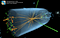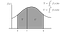# How do Transistors work?

## Almost like a flush toilet, indeed…

Transistors are the building blocks of our electronic gadgets: from smartphones to computers, toys, cooking devices, cleaning tools, etc.

Despite their ubiquity, only a few people know about how they work. In fact, understanding their principles of operation is not so hard.

# The diode: a transistor building block

In order to understand how a transistor work, we need to understand what a diode is. In fact, a transistor, in practice, is nothing but two diodes.

A diode, essentially, works as a valve for electric current. It lets a flow of electrons flow in one direction only, opposing the movement of electrons in the opposite direction, just as a water valve lets a flow of water flow in one direction only. …

# Salt on an Icy Road Lowers Its Temperature

## So, how does it melt the ice?

In a previous post of mine, I illustrated a brief history of temperature scales in which I recalled that the reason for which 0 °F corresponds to －18 °C is that the latter was the lowest temperature that Daniel Gabriel Fahrenheit could achieve in his laboratory.

Such a temperature can be easily reached mixing water, ice and ammonium chloride. This was, in fact, a very common method to produce a frigorific mixture before the invention of the freezer. The melting of the salts in water, in fact, is a chemical reaction that requires heat to proceed. Indeed, as every Italian knows, to prepare a good pasta dish you have to throw it in plenty of boiling salted water. Salt is usually put in the water when it has already reached the boiling point, otherwise it would take longer to reach this condition. …

# Temperature Scales

The most widely adopted temperature scale is the Celsius or centigrade scale. Nowadays, we use these terms as synonyms, but, in fact, they were not such, originally. The Celsius temperature scale was invented by Anders Celsius that, in 1742, proposed its use by publishing “Observationer om twänne beständiga grader på en thermometer” (Observations of two constant gradations on a thermometer).

In his paper, Celsius reported the observations, according to which the temperature of melting ice (marked as C) and that of boiling water (marked as D) appeared to be constant, if measured with a mercury thermometer.

He then proposed, on page 179, to divide the length between these two points into 100 parts and assigned the value 100 to point C (melting ice), saving the value 0 for point D (boiling water). …

# An Unorthodox Lecture on Electric Currents

## An introduction to the concept of an electrical current

Most physics textbooks are similar. They differ (little) for the sequence and for the choice of the mathematical details with which topics are presented. Most authors and teachers do not even ask themselves why they illustrate topics that way. Apparently, there is only one way to introduce something.

I believe this is not true. At least, I find stimulating trying to find alternative ways to introduce something in a different way. It is a difficult task, of course, and that is, maybe, why it is rewarding.

Let me be clear: I am not stating that alternative ways of teaching something are better than traditional ones. They are just different. Someone may appreciate them, some other may not. …

# Two Myths of Numerical Integration, Debunked

Many programmers believe that the use of higher order integration algorithms, combined with a large number of integration interval divisions, is useful (and sometimes necessary) to achieve good accuracy. In this article we show that this is not always true.

# Myth No. 1: Higher Order Methods Are Better

Browsing Cantor’s Paradise articles, one of my favourite publications on Medium, I found an article written by Kazi Abu Rousan, in which the use of the trapezoidal rule for numerical integration is advocated (I am attracted by articles about physics, numerical methods and programming, as well as about the interplay between art and science). It is a well written article, in fact (as usual, on this publication) and I appreciate very much the animated figures that clearly illustrate the method. …

# Understanding the Higgs Mechanism

## A non-metaphoric explanation

If you have an elementary knowledge of physics, it is very possible that you are unsatisfied about the metaphoric explanations of the role of the Higgs boson in “giving mass to other particles”. On the other hand we do not explain gravity and electromagnetism in a similar way. We teach them using mathematical equations, whose meaning is very clear and precise, and allow for the computation of measurable quantities.An event collected by the CMS collaboration at LHC showing a Higgs boson decaying into a pair of photons (the two long green towers, representing energy deposits into the photon detector)

In a recent post by Ethan Siegel, he correctly pointed it out that most of the mass of the Universe comes, in fact, from strong interactions inside nucleons (protons and neutrons), i.e., …

# Numerical integration: the wrong way

## How a small error may lead to a disaster

Numerical integration methods are those that allow the calculation of the definite integrals of a function, using computers. Simply stated, numerical integration is the process that leads to the evaluation, in many cases approximate, of the area between a curve y=f(x) and the x-axis, in a region x∈[a,b].The integral between a and c of the function f(x) represents the grey-shaded area T; the integral between c and b is the area indicated with U. The integral between a and b represents the sum of the areas T+U. Image taken from Wikimedia Commons (thanks to Juliusross~commonswiki).

One of the simplest and, at the same time, most widely used method of numerical integration dates back to the time of Isaac Newton (this demonstrates that numerical calculation is not at all, as is believed, closely related to the use of computers). It consists of dividing the interval [a,b] into a series of N smaller intervals [xᵢ,xᵢ₊₁], approximate f(x) in each of them with a constant f(x)≃f(xᵢ)=const, and approximate the area under the curve as the sum of the areas of rectangles whose base is xᵢ₊₁-xᵢ and whose height is f(x̅), where is the midpoint of the sub-interval [xᵢ,xᵢ₊₁]. …

# 11+3 tips to solve physics problems

## A how-to guide

Practically all the exercise books I have (publishers send me some from time to time) are all the same: only the set of exercises changes. The same exercise, in fact, can be dressed in a different way and, therefore, they are not so different either. I must confess that I do not like any of them.

Rather then teaching how to solve a problem, the chapters of these books usually open with a reference to the fundamental formulas concerning the subject dealt with, and then propose a series of exercises, of increasing complexity, followed by their solution, which are almost always presented in a very clean, plain, natural and obvious form. …

# The Fermi-Dirac distribution

## Enrico Fermi became famous, in the community of physicists of the time, for having published, in 1926, an article in which he calculated the energy distribution of identical particles with half integer spin. In the same year, but a little later, Paul Dirac independently came to the same result and for this reason the distribution is now called Fermi-Dirac. Here we illustrate its meaning.

Matter, as we know, is made up of electrons, neutrons and protons, which are are particles with half-integer spin, that is particles with an intrinsic angular momentum equal to half of an integer number (in this case equal to 1/2).

The intrinsic angular momentum (or spin) is often described as a classical intrinsic angular momentum, i.e. as a quantity that measures the speed of rotation of a particle around its axis. This picture is completely wrong, in fact. The spin has no classical counterpart and certainly cannot be thought of as a classical angular momentum: particles are point-like and do not rotate around any axis. It is better to think of it as a quantity that can be measured for these particles. They can then be labeled with the values +1/2 or −1/2, which determines, along with other quantities, their state. …

# Is 10 million times 7 equal to 70 million?

## Numerical computation can lead to subtle rounding errors. Good programmers must know how to avoid them.

Consider the following program, written in C:

`#include <stdio.h>int main() { float x = 7; float S = 0; int i; for (i = 0; i < 10000000; i++) {   S += x; } printf(“%f\n”, S);}`

The program adds 7 to the variable S ten million times. The expected result is thus 70 million. Indeed, compiling (with no optimisation) and running the program gives

`77603248.000000`

That is more than 10% larger. Surprised? Well, this is the effect of rounding errors caused by the fact that numbers, in computers, can only be represented with a finite number of digits. In fact, both 7 and 10 million are perfectly representable in a computer’s memory. …